NCERT Questions

Class 10
Chapter 12 Class 10 - Electricity

## (a) 1:2 (b) 2:1 (c) 1:4 (d) 4:1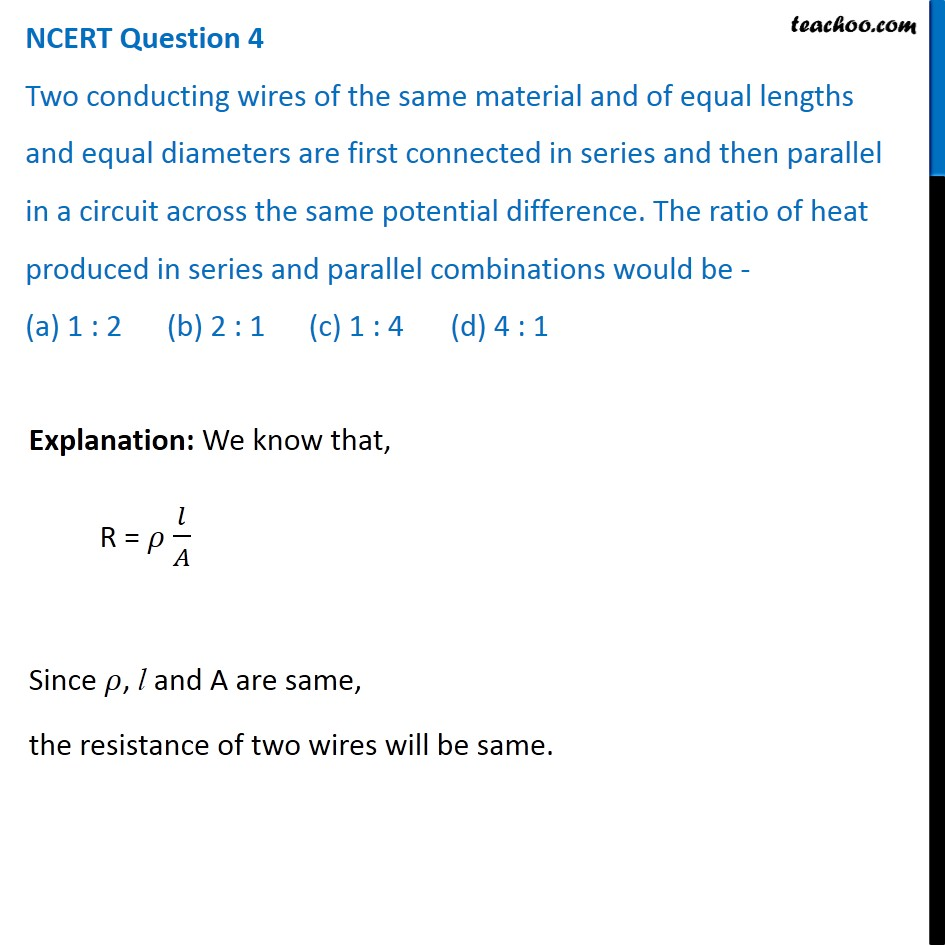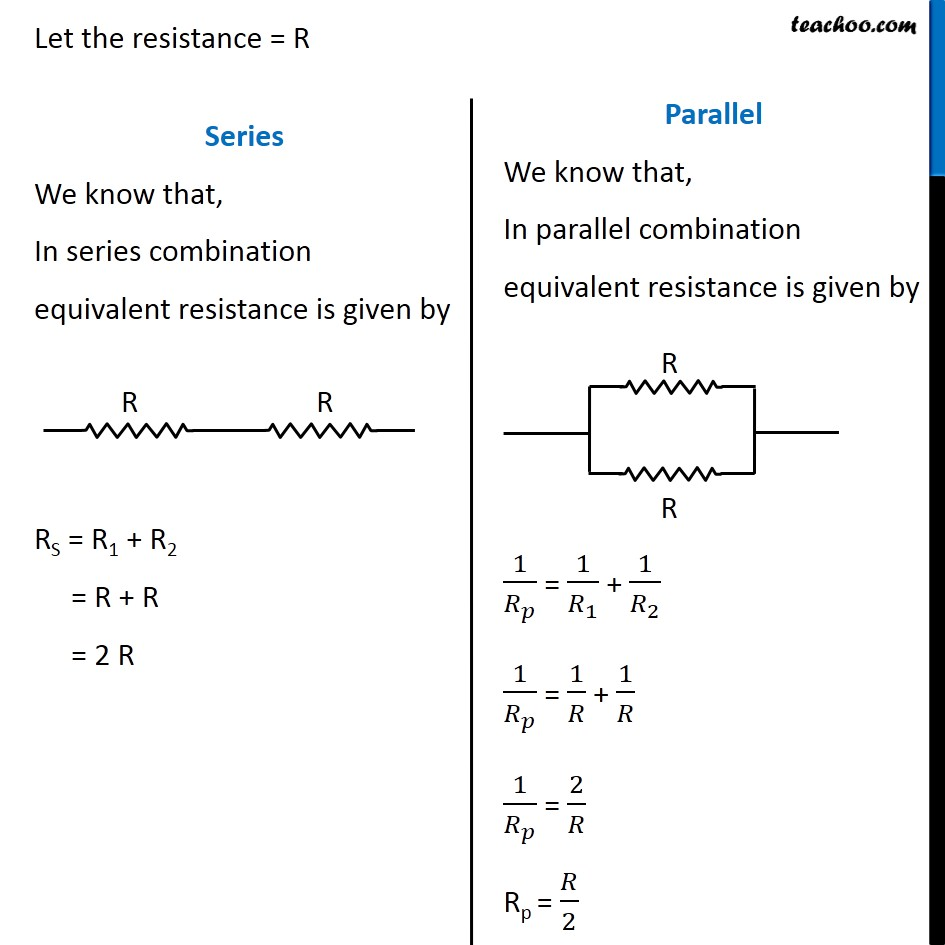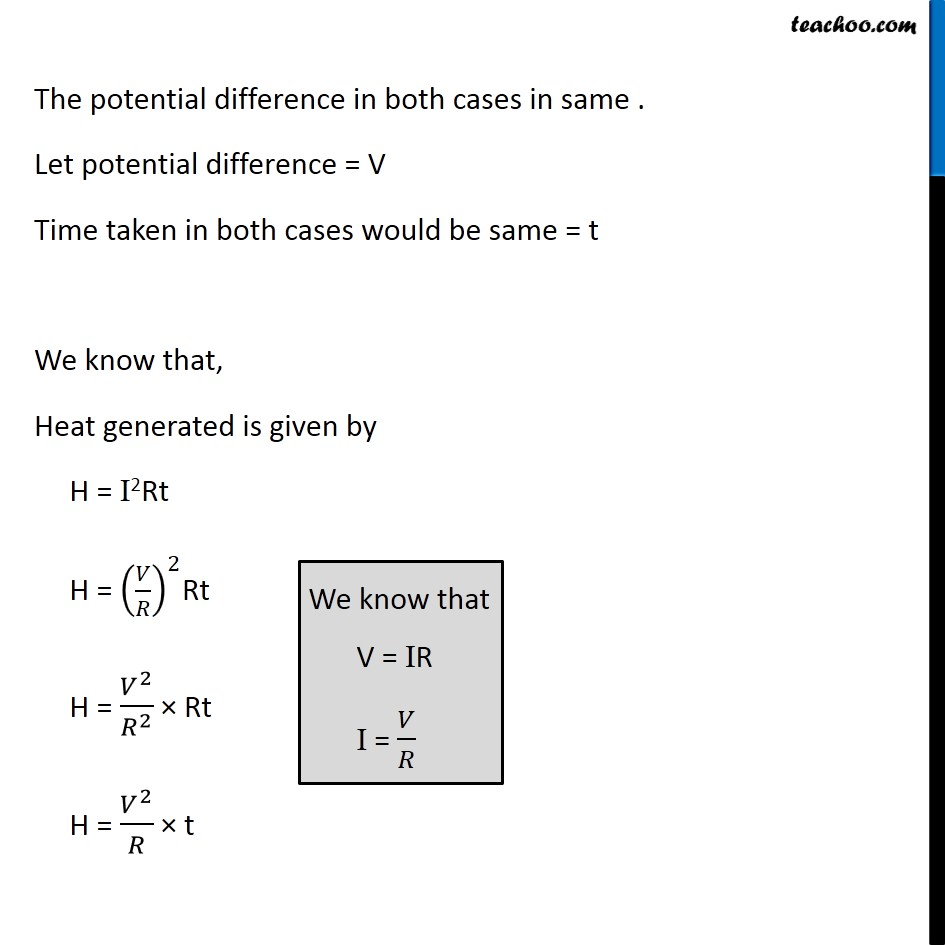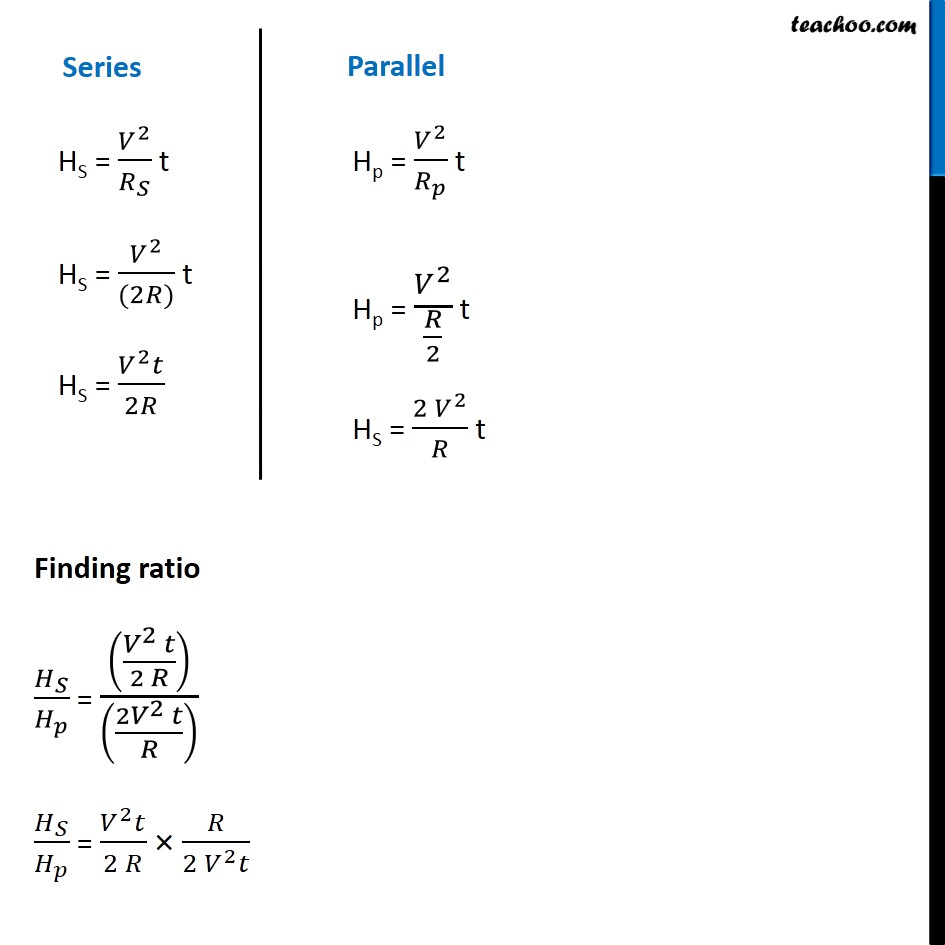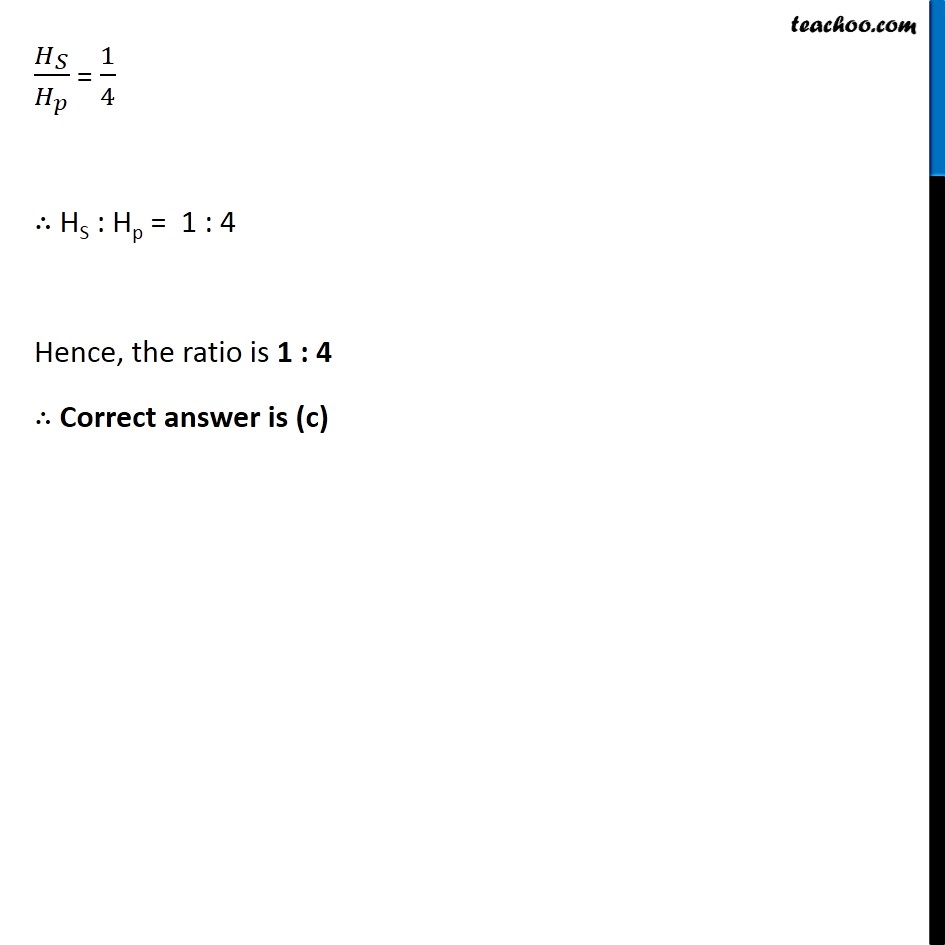Learn in your speed, with individual attention - Teachoo Maths 1-on-1 Class

### Transcript

NCERT Question 4 Two conducting wires of the same material and of equal lengths and equal diameters are first connected in series and then parallel in a circuit across the same potential difference. The ratio of heat produced in series and parallel combinations would be - (a) 1 : 2 (b) 2 : 1 (c) 1 : 4 (d) 4 : 1 Explanation: We know that, R = 𝜌 𝑙/𝐴 Since 𝜌, l and A are same, the resistance of two wires will be same. Let the resistance = R Series We know that, In series combination equivalent resistance is given by RS = R1 + R2 = R + R = 2 R Parallel We know that, In parallel combination equivalent resistance is given by 1/𝑅_𝑝 = 1/𝑅_1 + 1/𝑅_2 1/𝑅_𝑝 = 1/𝑅 + 1/𝑅 1/𝑅_𝑝 = 2/𝑅 Rp = 𝑅/2 The potential difference in both cases in same . Let potential difference = V Time taken in both cases would be same = t We know that, Heat generated is given by H = I2Rt H = (𝑉/𝑅)^2Rt H = 𝑉^2/𝑅^2 × Rt H = 𝑉^2/𝑅 × t We know that V = IR I = 𝑉/𝑅 Series HS = 𝑉^2/𝑅_𝑆 t HS = 𝑉^2/((2𝑅)) t HS = (𝑉^2 𝑡)/2𝑅 Parallel Hp = 𝑉^2/𝑅_𝑝 t Hp = 𝑉^2/(𝑅/2) t HS = (2 𝑉^2)/𝑅 t Finding ratio 𝐻_𝑆/𝐻_𝑝 = (((𝑉^2 𝑡)/(2 𝑅)))/(((2𝑉^2 𝑡)/𝑅) ) 𝐻_𝑆/𝐻_𝑝 = (𝑉^2 𝑡)/(2 𝑅) × 𝑅/(2 𝑉^2 𝑡) 𝐻_𝑆/𝐻_𝑝 = 1/4 ∴ HS : Hp = 1 : 4 Hence, the ratio is 1 : 4 ∴ Correct answer is (c)